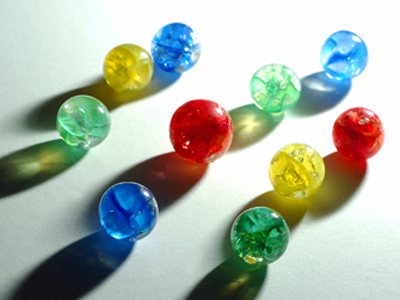The ratio of blue, green yellow and red marbles is 3:3:2:2.

# Ratio 2 (Difficult)

Ratios are an important part of the Eleven Plus maths exam and you will need to understand them if you want to do well. But you’ve come to the right place if you need more practice. This is the second quiz in our Difficult section on ratios.

We use ratios in a similar way to fractions. Both are ways to represent parts or portions of a larger number. For example, let’s say that John has 3 sweets and Jill has 2. We could show this as the ratio 3:2 or as the fractions 35 and 25. That’s because there are 5 sweets altogether. John has 3 of them and Jill has 2.

I hope that makes things clear. If not, don’t worry – you’ll get plenty of opportunity to practice in this quiz, and our others of ratios. Keep trying until you get all the questions right. Good luck!

1.
Adam, Beth and Carla are stamp collectors. Adam has 44 stamps, Beth has 68 and Carla has 76. What is the simplest ratio to express these amounts?
11:17:19
22:34:38
44:68:76
5:8:10
A:B:C: = 44:68:76 = 11:17:19 (dividing by 4)
2.
Andrew, Beatrice and Clair earned £700 for a job. They shared it in the ratio 4:3:7 respectively. How much did each person get?
Andrew £300, Beatrice £200 and Clair £200
Andrew £300, Beatrice £250 and Clair £150
Andrew £200, Beatrice £150 and Clair £350
Andrew £350, Beatrice £150 and Clair £200
4 + 3 + 7 = 14 ∴ the fractional parts are as follows:
Andrew, 414 × £700 = £200;
Beatrice, 314 × £700 = £150;
Clair, 714× £700 = £350
3.
Anna, Brian and Charles are given 17 sweets to share. The sweets are given out in the ratio 12:10:12 respectively. How many sweets did each child get?
Anna 12, Brian 10 and Charles 12
Anna 6, Brian 5 and Charles 6
Anna 8, Brian 7 and Charles 8
Anna 3, Brian 4 and Charles 3
12:10:12 can be simplified by dividing by two to make 6:5:6. This ratio adds up to 17 so that is the number of sweets each child received
4.
How else can the ratio 1.5:2:1.2 be written?
2:4:1
10:15:8
15:20:10
15:20:12
1.5:2:1.2 = 15:20:12 (multiplying by 10). You need to find a number to multiply by which will make 1.5 and 1.2 into whole numbers
5.
650 orders have been made for 3 products: TV sets, microwaves and fridges in the ratio 8:2:3 respectively. How many items of each product have been ordered?
400 TV sets, 100 microwaves and 150 fridges
80 TV sets, 20 microwaves and 30 fridges
450 TV sets, 150 microwaves and 200 fridges
160 TV sets, 40 microwaves and 120 fridges
8 + 2 + 3 = 13 ∴ the fractional parts are as follows: TVs, 813 × 650 = 400; microwaves, 213 × 650 = 100; fridges, 313 × 650 = 150
6.
How else can the ratio 2.5:1.25:7 be written?
10:5:28
10:4:28
11:5:28
10:5:24
2.5:1.25:7 = 10:5:28 (multiply by 4)
7.
How else can the ratio 4:1.2 be written?
3:10
20:5
20:7
10:3
4:1.2 = 20:6 (multiply by 5). This can be simplified to 10:3 by dividing by two
8.
What are the fractional parts of the ratio 5:9:3?
518, 918 and 318
517, 917 and 317
519, 919 and 319
520, 920 and 320
5 + 9 + 3 = 17 which becomes the denominator in the fractional parts
9.
What are the fractional parts of 7:4:11?
711, 411 and 1111
722, 422 and 1122
13, 15 and 12
1422, 822 and 2222
7 + 4 + 11 = 22 which becomes the denominator in the fractional parts
10.
What are the fractional parts of the ratio 3:18 in their simplest form?
16 and 66
121 and 621
17 and 67
321 and 1821
3 + 18 = 21. This can be simplified by dividing by three to 1 + 6 = 7 which becomes the denominator in the fractional parts. Always try to simplify if it is possible
Author:  Frank Evans